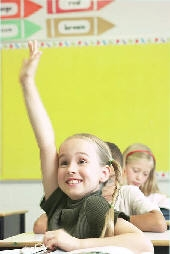This area contains resources and activities covering core curriculum learning areas for children in elementary school, grades 1 – 6.  Select from the subjects on the left to browse for learning activities you can do with your particular age group.# Standard Elementary School Curriculum Guidelines

Here are some rough guidelines for what children typically learn at different grades throughout elementary school:

• Typically includes recognizing double digit numbers
• Beginning to learn addition and subtraction concepts using objects
• Beginning reading using simple sentences

• Single digit addition and subtraction
• Learning how to tell time

• Continue addition and subtraction using double digit or multiple digit numbers
• Beginning multiplication
• Start cursive writing (though many school are doing away with this)

• Identify and use fractions and decimals, and compare them on a number line
• Handling money and making change
• Beginning division
• Focus shifts from learning to read to reading to learn, which means kids who aren’t proficient readers by this time start to fall farther behind

• Be proficient at multiplication & division
• Be able to do math comparing fractions and decimals and common percents, adding and subtracting them
• Solve problems involving perimeter and area of triangles and all quadrilaterals having at least one pair of parallel sides (ie trapezoids)
• Dissection  and biology